• 文中选用2006年,2013年,2018年三期Landsat卫星影像来对泰安市进行研究,运用ENVI5.3对环境影响因子(干度,绿度,湿度,热度)进行提取并进行分析处理,对得到的遥感生态指数(RSEI)综合分析来评价泰安市生态质量。...
• 利用平朔矿区1989—2018年4个时相的Landsat( 影像，运用主成分分析法，集成绿度（NDVI）、湿度（WET）、干度（NDSI）和热度（LST）4项指标构建遥感生态指数（RSEI）综合评估模型，对平朔矿区的生态环境状况进行综合...
• 遥感生态指数、 RSEI、 水体掩膜、 主成分分析、 PCA、 Landsat、 GEE、 gee、

目录
RSEI原理
湿度指标（Wet）
绿度指标（NDVI）
热度指标（LST）
干度指标（NDBSI）
Landsat-8波段
归一化（normalization）
主成分分析( PCA )
PCA-->RSEI
Wet、NDVI、LST、NDBSI、PCA、RSEI之间的关系
代码
运行结果
感谢

RSEI原理

RSEI是一个完全基于遥感技术，以自然因子为主的遥感生态指数(RSEI) 来对城市的生态状况进行快速监测与评价的方法，由徐涵秋.城市遥感生态指数的创建及其应用[J].生态学报,2013,33(24):7853-7862.中提出。
该指数利用主成分分析技术集成了植被指数、湿度分量、地表温度和建筑指数等 4 个评价指标，它们分别代表了绿度、湿度、热度和干度等 4 大生态要素。

湿度指标（Wet）

WET = c1 B1 + c2 B2 + c3 B3 + c4 B4 + c5 B5 + c6 B6
B1-B6 分别代表蓝波段、绿波段、红波段、近红波段、中红外波段 1、中红外波段 2；
c1~c6是传感器参数。由于传感器的类型不同，参数也相应有所不同。
其中，TM 传感器，c1 ～ c6 分 别 为 0.0315、0.2021、0.3012、0.1594、-0.6806、-0.6109；
OLI 传感器，c1 ～ c6 分别为 0.1511、 0.1973、 0.3283、 0.3407、-0.7117、 -0.4559。
Landsat 8上携带的是陆地成像仪（Operational Land Imager ，OLI）

绿度指标（NDVI）

NDVI=（NIR-Red）/（NIR+Red）

热度指标（LST）
直接计算有点麻烦，故直接采用 MODIS 的LST，利用以下公式
LST = （ LST_Day_1km + LST_Night_1km ）/ 2

补充：现在才知道 Landsat 也有LST产品，且分辨率为30m，但是不改代码了，如何分辨率改为30m，下面代码的研究区太大了，会有点问题
LANDSAT/LC08/C02/T1_L2
介绍：
https://developers.google.com/earth-engine/datasets/catalog/LANDSAT_LC08_C02_T1_L2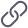https://developers.google.com/earth-engine/datasets/catalog/LANDSAT_LC08_C02_T1_L2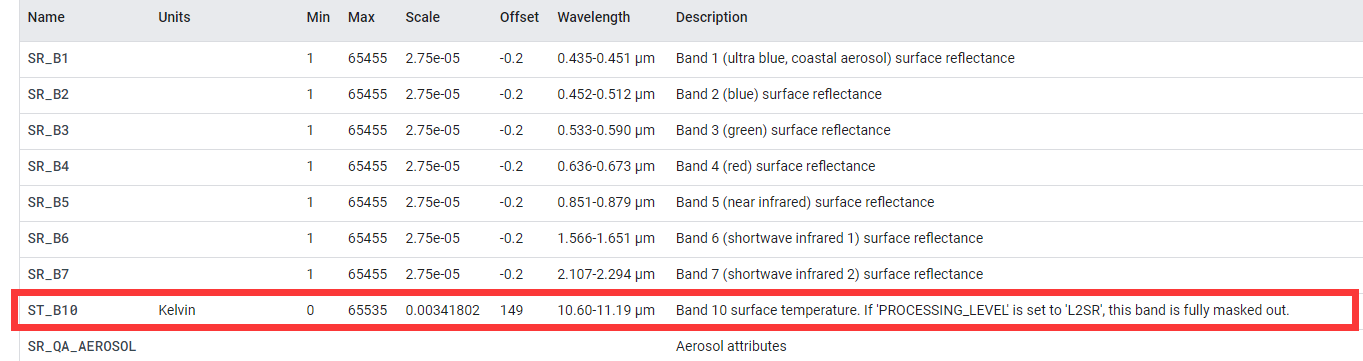干度指标（NDBSI）

IBI 是建筑指数
SI为土壤指数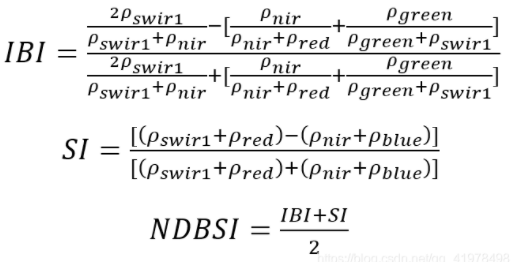Landsat-8波段

徐涵秋使用的是Landsat-7 ETM+遥感影像，但是本文采用的是Landsat-8遥感影像为例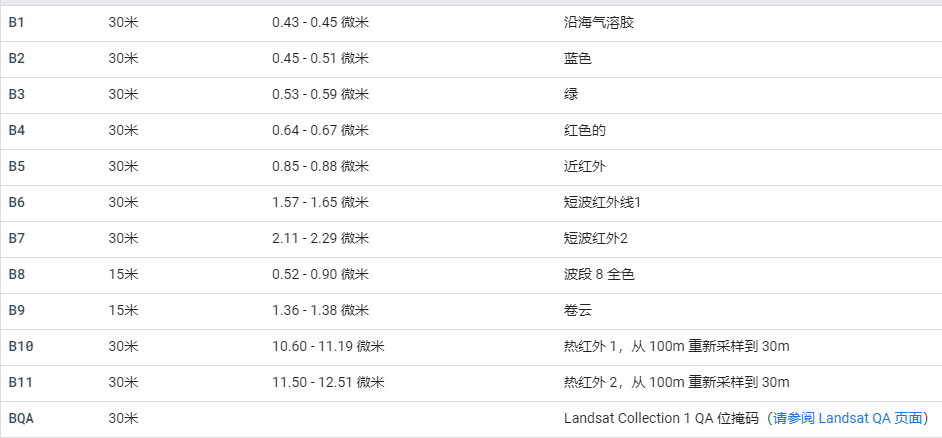归一化（normalization）

先使用ee.Reducer.minMax求出该幅遥感影像的最大值和最小值
在使用unitScale(low, high)使输入值的范围[低，高]变为[0，1]

主成分分析( PCA )

PCA就是一个有损的降维算法。
具体的请看代码的英文注释，不敢讲，请自行百度理解。
推荐
GEE主成分分析全流程Google earth engine如何进行主成分分析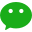https://mp.weixin.qq.com/s/spi10OeHDRo6VpIrrpvYHA

PCA-->RSEI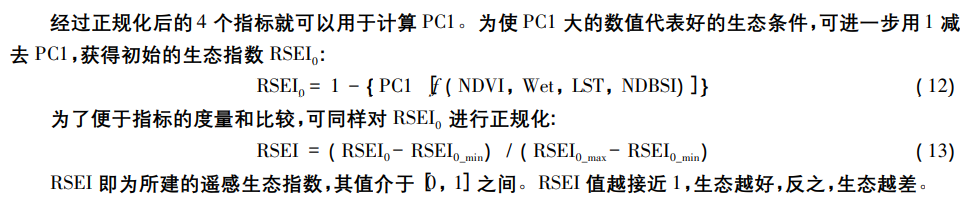Wet、NDVI、LST、NDBSI、PCA、RSEI之间的关系

在 PC1 中，代表绿度的 NDVI 和代表湿度的 Wet 指标呈正值，说明它们对生态系统起 正面的贡献，而代表热度和干度的 LST、NDBSI 则呈负值，这与实际情况相符。
综合来看，以 NDVI 为代表的植被和以 NDBSI 为代表的建筑用地对城市生态的影响力最大，且 NDVI 大 于 NDBSI。NDBSI 所代表的建筑不透水面对城市地表温度LST具有正相关关系
RSEI 即为所建的遥感生态指数，其值介于［0，1］之间。ＲSEI 值越接近 1，生态越好，反之，生态越差。
好好品一下这个话：为使 PC1 大的数值代表好的生态条件，可进一步用 1 减 去 PC1，获得初始的生态指数 RSEI0
PC1的值大，不代表生态好，PC1小的值，也不代表生态不好。只有RSEI0 = 1 - PC1才能代表生态

代码

// roi和table不是一个geometry，运行的话，注意一下
var roi = table.geometry().bounds()

Map.centerObject(roi, 5);

function remove_water(img) {
var year = img.get('year')
// jrc_year: JRC Yearly Water Classification History, v1.3
.filterBounds(roi)
.first()
.select('waterClass')
.reproject('EPSG:4326',null,1000)
// 掩膜掉大片永久水体
.eq(3)
}

function removeCloud(image){
var qa = image.select('BQA')
var cloudMask = qa.bitwiseAnd(1 << 4).eq(0)
}

var L8 = ee.ImageCollection('LANDSAT/LC08/C01/T1_TOA')
.filterBounds(roi)
.filterDate('2014-01-01', '2019-12-31')
.map(function(image){
return image.set('year', ee.Image(image).date().get('year'))
})
.map(removeCloud)

var L8imgList = ee.List([])
for(var a = 2014; a < 2020; a++){
var img = L8.filterMetadata('year', 'equals', a).median().clip(roi)
var L8img = img.set('year', a)
}

var L8imgCol = ee.ImageCollection(L8imgList)
.map(function(img){
return img.clip(roi)
})
.map(remove_water)

L8imgCol = L8imgCol.map(function(img){

// Wet
var Wet = img.expression('B*(0.1509) + G*(0.1973) + R*(0.3279) + NIR*(0.3406) + SWIR1*(-0.7112) + SWIR2*(-0.4572)',{
'B': img.select(['B2']),
'G': img.select(['B3']),
'R': img.select(['B4']),
'NIR': img.select(['B5']),
'SWIR1': img.select(['B6']),
'SWIR2': img.select(['B7'])
})

// NDVI
var ndvi = img.normalizedDifference(['B5', 'B4']);

// lst 直接采用MODIS产品，表示看不懂 LST 公式
var lst = ee.ImageCollection('MODIS/006/MOD11A1').map(function(img){
return img.clip(roi)
})
.filterDate('2014-01-01', '2019-12-31')

var year = img.get('year')
lst=lst.filterDate(ee.String(year).cat('-01-01'),ee.String(year).cat('-12-31')).select(['LST_Day_1km', 'LST_Night_1km']);

// reproject主要是为了确保分辨率为1000
var img_mean=lst.mean().reproject('EPSG:4326',null,1000);
//print(img_mean.projection().nominalScale())

img_mean = img_mean.expression('((Day + Night) / 2)',{
'Day': img_mean.select(['LST_Day_1km']),
'Night': img_mean.select(['LST_Night_1km']),
})

// ndbsi = ( ibi + si ) / 2
var ibi = img.expression('(2 * SWIR1 / (SWIR1 + NIR) - (NIR / (NIR + RED) + GREEN / (GREEN + SWIR1))) / (2 * SWIR1 / (SWIR1 + NIR) + (NIR / (NIR + RED) + GREEN / (GREEN + SWIR1)))', {
'SWIR1': img.select('B6'),
'NIR': img.select('B5'),
'RED': img.select('B4'),
'GREEN': img.select('B3')
})
var si = img.expression('((SWIR1 + RED) - (NIR + BLUE)) / ((SWIR1 + RED) + (NIR + BLUE))', {
'SWIR1': img.select('B6'),
'NIR': img.select('B5'),
'RED': img.select('B4'),
'BLUE': img.select('B2')
})
})

var bandNames = ['NDVI', "NDBSI", "WET", "LST"]
L8imgCol = L8imgCol.select(bandNames)

// 归一化
var img_normalize = function(img){
var minMax = img.reduceRegion({
reducer:ee.Reducer.minMax(),
geometry: roi,
scale: 1000,
maxPixels: 10e13,
})
var year = img.get('year')
var normalize  = ee.ImageCollection.fromImages(
img.bandNames().map(function(name){
name = ee.String(name);
var band = img.select(name);
return band.unitScale(ee.Number(minMax.get(name.cat('_min'))), ee.Number(minMax.get(name.cat('_max'))));

})
).toBands().rename(img.bandNames()).set('year', year);
return normalize;
}
var imgNorcol  = L8imgCol.map(img_normalize);

// 主成分
var pca = function(img){

var bandNames = img.bandNames();
var region = roi;
var year = img.get('year')
// Mean center the data to enable a faster covariance reducer
// and an SD stretch of the principal components.
var meanDict = img.reduceRegion({
reducer:  ee.Reducer.mean(),
geometry: region,
scale: 1000,
maxPixels: 10e13
});
var means = ee.Image.constant(meanDict.values(bandNames));
var centered = img.subtract(means).set('year', year);

// This helper function returns a list of new band names.
var getNewBandNames = function(prefix, bandNames){
var seq = ee.List.sequence(1, 4);
//var seq = ee.List.sequence(1, bandNames.length());
return seq.map(function(n){
return ee.String(prefix).cat(ee.Number(n).int());
});
};

// This function accepts mean centered imagery, a scale and
// a region in which to perform the analysis.  It returns the
// Principal Components (PC) in the region as a new image.
var getPrincipalComponents = function(centered, scale, region){
var year = centered.get('year')
var arrays = centered.toArray();

// Compute the covariance of the bands within the region.
var covar = arrays.reduceRegion({
reducer: ee.Reducer.centeredCovariance(),
geometry: region,
scale: scale,
bestEffort:true,
maxPixels: 10e13
});

// Get the 'array' covariance result and cast to an array.
// This represents the band-to-band covariance within the region.
var covarArray = ee.Array(covar.get('array'));

// Perform an eigen analysis and slice apart the values and vectors.
var eigens = covarArray.eigen();

// This is a P-length vector of Eigenvalues.
var eigenValues = eigens.slice(1, 0, 1);
// This is a PxP matrix with eigenvectors in rows.
var eigenVectors = eigens.slice(1, 1);

// Convert the array image to 2D arrays for matrix computations.
var arrayImage = arrays.toArray(1)
// Left multiply the image array by the matrix of eigenvectors.
var principalComponents = ee.Image(eigenVectors).matrixMultiply(arrayImage);

// Turn the square roots of the Eigenvalues into a P-band image.
var sdImage = ee.Image(eigenValues.sqrt())
.arrayProject().arrayFlatten([getNewBandNames('SD',bandNames)]);

// Turn the PCs into a P-band image, normalized by SD.
return principalComponents
// Throw out an an unneeded dimension, [[]] -> [].
.arrayProject()
// Make the one band array image a multi-band image, [] -> image.
.arrayFlatten([getNewBandNames('PC', bandNames)])
// Normalize the PCs by their SDs.
.divide(sdImage)
.set('year', year);
}

// Get the PCs at the specified scale and in the specified region
img = getPrincipalComponents(centered, 1000, region);
return img;
};

var PCA_imgcol = imgNorcol.map(pca)

// 利用PC1，计算RSEI，并归一化
var RSEI_imgcol = PCA_imgcol.map(function(img){
var rsei = img.expression('constant - pc1' , {
constant: img.select('constant'),
pc1: img.select('PC1')
})
rsei = img_normalize(rsei)
})
print(RSEI_imgcol)

var visParam = {
palette: 'FFFFFF, CE7E45, DF923D, F1B555, FCD163, 99B718, 74A901, 66A000, 529400,' +
'3E8601, 207401, 056201, 004C00, 023B01, 012E01, 011D01, 011301'
};

//Foo.foo(rsei_imgcol.first(), roi);
运行结果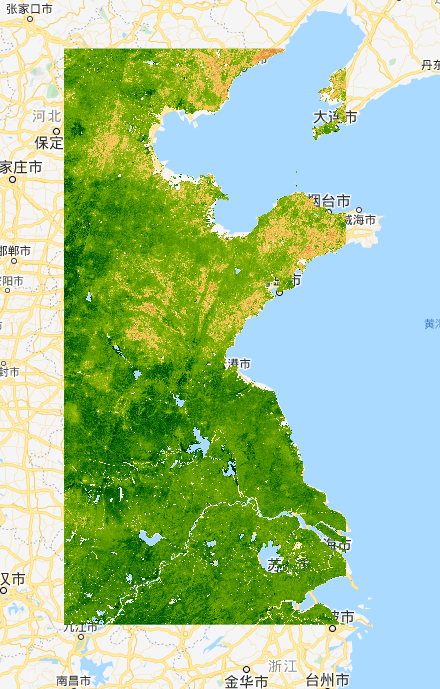感谢
遥感生态指数（RSEI）——四个指数的计算_系六九69的博客-CSDN博客_rsei
利用GEE逐年计算1990-2020年秦岭北麓遥感生态指数（RSEI）二 - 知乎https://zhuanlan.zhihu.com/p/347934313

展开全文主成分分析 PCA
• 遥感生态指数是在4个生态指数的基础上通过主成分变换得出的一个评价生态环境质量的指标。因此，第二部分就是要分别计算出这4个指数的值。 ①首先，是绿度指数的计算，一般用归一化植被指数（NDVI） NDVI=（NIR-...
遥感生态指数是在4个生态指数的基础上通过主成分变换得出的一个评价生态环境质量的指标。因此，第二部分就是要分别计算出这4个指数的值。
①首先，是绿度指数的计算，一般用归一化植被指数（NDVI）
NDVI=（NIR-Red）/（NIR+Red）
在Bandmath工具中输入NDVI计算公式  (float(b4-b3))/(b4+b3)  其中，b3、b4分别是红波段和近红外波段
②其次，是湿度计算。
WET = c1B1 + c2B2 + c3B3 + c4B4 + c5B5 + c6B6
B1-B6 分别代表蓝波段、绿波段、红波段、近红波段、中红外波段 1、中红外波段 2；c1~c6是传感器参数。由于传感器的类型不同，参数也相应有所不同。其中，TM 传感器，c1 ～ c6 分 别 为 0.0315、0.2021、0.3012、0.1594、-0.6806、-0.6109； OLI 传感器，c1 ～ c6 分别为 0.1511、 0.1973、 0.3283、 0.3407、-0.7117、 -0.4559。
故，在Bandmath中，输入湿度的计算公式：
Wet-TM= (b1*0.0315+b2*0.2021+b3*0.3012+b4*0.1594+b5*(-0.6806)+b7*(-0.6109))/10000
Wet-OLT=(b1*0.1511+b2*0.1973+b3*0.3283+b4*0.3407+b5*(-0.7117)+b7*(-0.4559))/10000
之所以在计算湿度过程中乘以1/10000，是为了使湿度的范围值落在[-1,1]之间。
③干度指数（NDBSI）计算。
干度指数（NDBSI）由城市建筑指数（IBI）和裸土指数（SI）的平均值得到的，该指数的范围是[-1,1]，值越大，表示越干燥。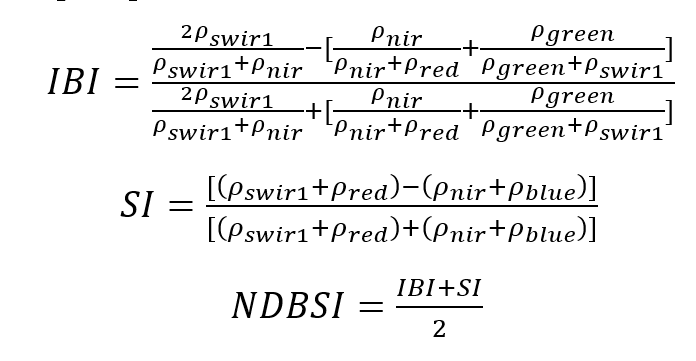其中，ρblue、ρgreen、ρred、ρnir、ρswir1分别表示蓝、绿、红、近红外、中红外1
在Bang math中输入公式为：
SI = (float((b3+b5)-(b1+b4)))/((b3+b5)+(b1+b4))
IBI=((float(2*b5))/(float(b5+b4))-((float(b4))/(float(b4+b3))+(float(b2))/(float(b2+b5))))/((float(2*b5))/(float(b5+b4))+                              ((float(b4))/(float(b4+b3))+(float(b2))/(float(b2+b5))))
其中，b1~b5分别为蓝、绿、红、近红外波段、中红外波段1.
NDSI = （b1+b2)/2   其中，b1、b2分别为IBI图像、SI图像。
④热度指数计算（LST）
使用大气矫正法对landsat-8地表温度进行反演 原理：首先估计大气对地表热辐射的影响, 然后把这部分大气影响从卫星传感器所观测到的热辐射总量中减去, 从而得到地表热辐射强度, 再把这一热辐射强度转化为相应的地表温度。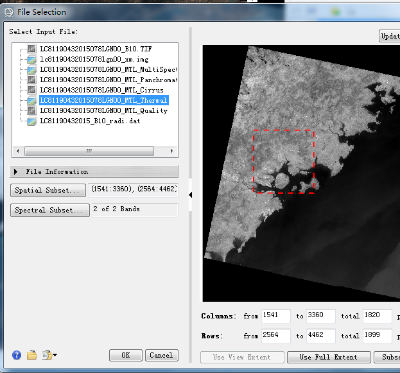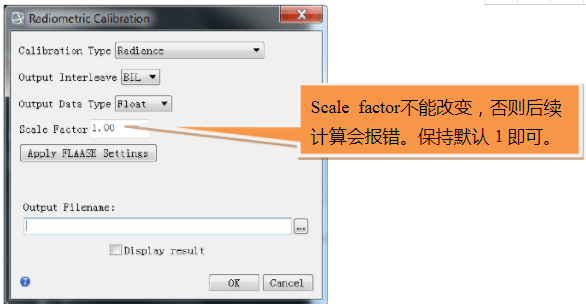2）计算NDVI
NDVI=(近红外-红)/(近红外+红)
3）计算植被覆盖度Fv
计算植被覆盖度Fv采用的是混合像元分解法，将整景影像的地类大致分为水体、植被和建筑，具体的计算公式如下：
FV = (NDVI- NDVIS)／(NDVIV - NDVIS)
其中，NDVI为归一化差异植被指数，取NDVIV = 0.70和NDVIS = 0.00，且有，当某个像元的NDVI大于0.70时，FV取值为1；当NDVI小于0.00，FV取值为0。
利用ENVI的Band Math，在公式输入栏中输入：
Fv=(b1 gt 0.7)*1+(b1 lt 0.05)*0+(b1 ge 0.05 and b1 le 0.7)*((b1-0.05)/(0.7-0.05))
其中b1为NDVI结果
4）地表比辐射率ε计算ε
根据前人的研究，将遥感影像分为水体、城镇和自然表面3种类型。本专题采取以下方法计算研究区地表比辐射率：水体像元的比辐射率赋值为0.995，自然表面和城镇像元的比辐射率估算则分别根据下式进行计算：
Ε(surface)=0.9625+0.0614*Fv-0.0461*FV2
Ε(building)=0.9589+0.086*Fv-0.0671*Fv2
式中，εsurface和εbuilding分别代表自然表面像元和城镇像元的比辐射率。
在ENVI中的计算公式：
Surf=(b1 le 0)*0.995+(b1 gt 0 and b1 lt 0.7)*(0.9589+0.086*b2-0.0671*b2*b2)+(b1 ge 0.7)*(0.9625+0.0614*b2-0.0461*b2*b2)
其中，b1为NDVI值，b2为植被覆盖度
5）计算相同温度下黑体的辐射亮度值
查询大气剖面数据(http://atmcorr.gsfc.nasa.gov/)，输入相关参数可得到大气剖面信息：大气在热红外波段的透过率（t），大气向上辐射亮度（Lu），大气向下辐射亮度（Ld）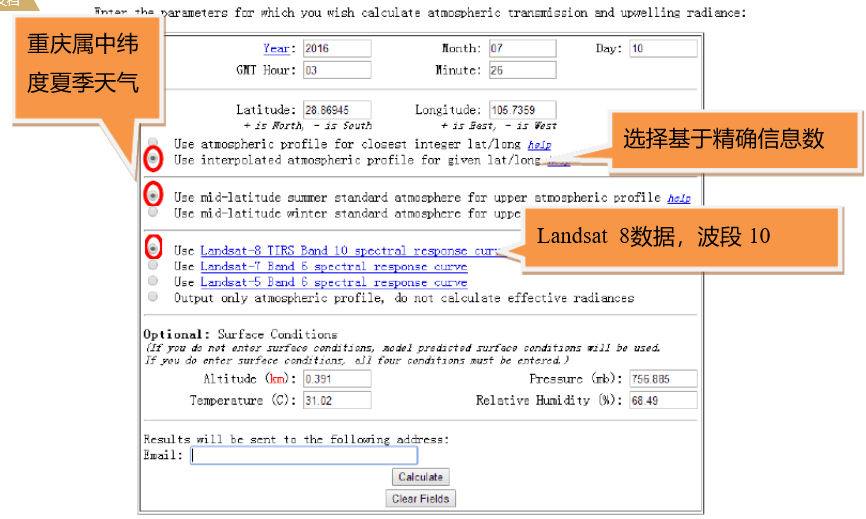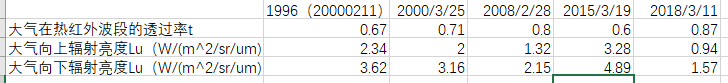BlackT=(b2-Lu-t*(1-b1)*Ld)/(t*b1)
2018：BlackT=(b2-0.94-0.87*(1-b1)*1.57)/(0.87*b1)
其中，b1选择地表比辐射率图像，b2选热红外波段辐射亮度图像
6）反演地表温度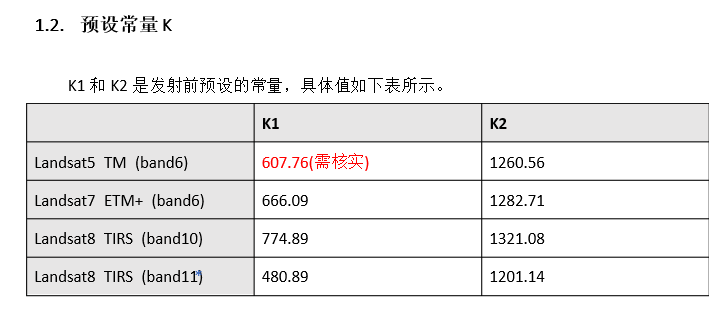Landsat 5：T=(1260.56)/alog(607.76/b1+1)-273
Landsat 8：T=(1321.08)/alog(774.89/b1+1)-273
其中，B1选择相同温度下黑体辐射亮度图像
至此，初步完成四个生态指数的计算。
展开全文• 最近在做一些遥感相关的图像处理项目，涉及到遥感生态指数的计算。由于项目要求Python实现，搜索互联网关于Python实现的遥感生态指数计算程序资料很少，于是就自己实现了一个并分享在这里，供需要的朋友参考。 首先...
最近在做一些遥感相关的图像处理项目，涉及到遥感生态指数的计算。由于项目要求Python实现，搜索互联网关于Python实现的遥感生态指数计算程序资料很少，于是就自己实现了一个并分享在这里，供需要的朋友参考。 首先需要了解遥感生态指数是什么，不是很清楚的朋友可以参考下面的几篇文章： 城市遥感生态指数的创建及其应用 区域生态环境变化的遥感评价指数 基于遥感生态指数的南京市生态变化分析 知道了遥感生态指数是什么后，程序实现其实比较简单，这里直接贴出程序。
"""
利用湿度、绿度、热度、干度计算遥感生态指数
"""
import os
import time
import numpy as np
from scipy import misc
from osgeo import gdal, gdalconst
from sklearn.decomposition import PCA

class CalIntegratedEcoIndex:

def __init__(self, img_path, res_save_dir):
self.deno_bias = 0.00001  # 分母偏置，防止除0
self.res_save_dir = res_save_dir

"""读取遥感数据信息"""

img_width = dataset.RasterXSize
img_height = dataset.RasterYSize
img_data = np.array(dataset.ReadAsArray(0, 0, img_width, img_height), dtype=float)  # 将数据写成数组，对应栅格矩阵

del dataset

return img_width, img_height, img_data

def get_wet_degree(self):
"""获取湿度指标"""
return 0.2626 * self.img + 0.2141 * self.img + 0.0926 * self.img + \
0.0656 * self.img - 0.7629 * self.img - 0.5388 * self.img

def get_green_degree(self):
"""获取绿度指标"""
return (self.img - self.img) / (self.img + self.img + self.deno_bias)

def get_temperature(self):
"""获取热度指标"""
gain = 3.20107  # landsat5 第6波段的增益值
bias = 0.25994  # 第6波段的偏置值

K1 = 606.09
K2 = 1282.71
_lambda = 11.45
_rho = 1.438e10-2
_epsilon = 0.96  # 比辐射率

DN = self.img * 299/1000 + self.img * 587/1000 + self.img * 114/1000  # 获取象元灰度值

L6 = gain * DN + bias
T = K2 / np.log(K1 / L6 + 1)
LST = T / (1 + (_lambda * T / _rho) * np.log(_epsilon))

return LST

def get_dryness_degree(self):
"""获取干度指标"""
band5_plus_band3 = self.img + self.img
band4_plus_band1 = self.img + self.img

SI = (band5_plus_band3 - band4_plus_band1) / (band5_plus_band3 + band4_plus_band1 + self.deno_bias)

left_expr = 2 * self.img / (self.img + self.img + self.deno_bias)
right_expr = self.img / (self.img + self.img + self.deno_bias) + \
self.img / (self.img + self.img + self.deno_bias)
IBI = (left_expr - right_expr) / (left_expr + right_expr + self.deno_bias)

NDBSI = (IBI + SI) / 2

return NDBSI

def arr2img(self, save_path, arr):
misc.imsave(save_path, arr)

def normlize(self, img_arr):
arr = np.array(img_arr)
return (arr - arr.min()) / (arr.max() - arr.min())

def get_rsei(self):
"""获取遥感生态指数"""
wet = self.get_wet_degree()
ndvi = self.get_green_degree()
lst = self.get_temperature()
ndbsi = self.get_dryness_degree()

data = np.array([self.normlize(wet), self.normlize(ndvi), self.normlize(lst), self.normlize(ndbsi)])
data = data.reshape(data.shape, -1).T

pca = PCA(n_components=1)
rsei = self.normlize(1 - np.reshape(pca.fit_transform(data), newshape=(self.img_height, self.img_width)))

return wet, ndvi, lst, ndbsi, 1 - rsei

def save_result(self, wet, ndvi, lst, ndbsi, rsei):
"""将各指数结果保存为图片"""
res_dict = {"wet": wet, "green": ndvi, "temperature": lst, "dry": ndbsi, "rsei": rsei}

for k, v in res_dict.items():
self.arr2img(os.path.join(self.res_save_dir, k + ".jpg"), v)

def get_average_index(self, wet, ndvi, lst, ndbsi, rsei):
"""获取归一化后各指标的平均值"""
return np.mean(self.normlize(wet)), np.mean(self.normlize(ndvi)), \
np.mean(self.normlize(lst)), np.mean(self.normlize(ndbsi)), np.mean(self.normlize(rsei))

程序可以按照下面的方式进行使用（注意输入图像是Landsat七波段的图像）：
if __name__ == '__main__':
start = time.time()
cal = CalIntegratedEcoIndex("/your/path/to/Landsat/image", "./result")
wet, ndvi, lst, ndbsi, rsei = cal.get_rsei()
aver_wet, aver_ndvi, aver_lst, aver_ndbsi, aver_rsei = cal.get_average_index(wet, ndvi, lst, ndbsi, rsei)
cal.save_result(wet, ndvi, lst, ndbsi, rsei)
end = time.time()

print("归一化后各指数的平均值\n湿度", aver_wet, "绿度", aver_ndvi, "热度", aver_lst, "干度", aver_ndbsi,
"遥感生态指数", aver_rsei, "Total cost time %.2f s" % (end - start))

因为对遥感领域不很了解，这也是我的初次尝试。如果程序中有什么错误及不足，欢迎读者朋友们在评论区批评指正。
展开全文图像处理 Python
• ①利用水体指数来增强水体信息，弱化其他地物的信息，便于提取研究区的水体范围 NDWI = (Green - NIR)/(Green + NIR ) MNDWI = (Green - SWIR1) / (Green + SWIR1) INDVI = (Red - NIR) / (Red + NIR) 以上三个...
1、水体掩膜
①利用水体指数来增强水体信息，弱化其他地物的信息，便于提取研究区的水体范围
NDWI = (Green - NIR)/(Green + NIR )
MNDWI = (Green - SWIR1) / (Green + SWIR1)
INDVI = (Red - NIR) / (Red + NIR)
以上三个水体指数都能在一定程度上突出水体，推荐使用MNDWI指数进行水体范围的提取。在Bandmath中输入水体指数法的计算公式：(float(b2-b5))/(b2+b5)，其中b2、b5分别表示绿光波段和中中红外1波段。得到增强水体后的图像，如图1

图1 增强水体

②在生成的水体指数图层，右击选择【New Raster Color Slice】，仅保存一个color，根据水体值的范围设置合理的color范围。一般情况下，水体的范围在[0.25,1]，具体的要根据研究区确定。我的研究区范围是厦门，水体范围大致在[0.4,1]之间，如图2、图3所示

图2 设置水体的范围值

图3 水体覆盖范围

③提取水体的范围，将该Slice导出为.shp格式

图4 将水体范围导出成shp矢量格式

图5 选择shp作掩膜区

图6 在option选择掩膜区是否参与计算

⑤根据制作的掩膜区对数据进行水体掩膜处理，在bandmath中输入公式 b1*b2 ，b1、b2分别选择掩膜区和待掩膜数据。由于不参与计算的区域值仍未0，在后续的主成分分析中仍然会参与进去，我一般情况下会把不参与计算的水面和非研究值设置成NaN，可以根据公式 (float(b1)*b1)/b1，将值为0的区域设置成Not a number
2、分别对NDVI、Wet、NDBSI、LST进行掩膜处理、数据标准化处理（归一化工具 Streatch data，范围为[0,1]）后，通过【Layer stacking】将以上四幅影像按NDVI、Wet、NDBSI、LST的顺序合成一景
3、主成分分析。【Transform】|【Principal Component】|【Forward PC Rotation】}【Compute New Statistics Rotate】。
一般情况下，第一主成分的贡献度占比超过80%，之后依次递减。

图7 各主成分的贡献度

4、经过主成分分析之后，第一主成分PC1即为初始遥感生态指数RSEI0。在个别情况下，生态较好的区域RSEI0值反而越低，这时候可以用 1-RSEI0 公式进行计算，使RSEI值高的地方表示生态越好。
5、对得到的RSEI值进行标准化处理，使得其范围在[0,1]之间。值越大，表示该地区的生态环境越好。
6、根据RSEI值对研究区的生态环境进行生态环境等级划分。图9是2018年厦门的遥感生态指数等级划分图（厦门的生态环境还是杠杠的~)

图8 生态环境等级划分

图9 来张结果图吧~

至此，完成遥感生态指数RSEI的计算啦 (说的容易，做起来，不容易哇！！）
当然，遥感生态指数不仅仅是计算RSEI这个指数啦，更重要的是对生态环境进行动态监测，就是在RSEI的基础上，对不同年份的遥感生态指数进行对比、分析，达到动态评价的效果~~
emm，这一篇写的好乱吼~大概只有我能看懂了（TT），就这样子吧，记录一下下~~
展开全文• 遥感生态指数（RSEI）是通过主成分耦合绿度、干度、湿度、热度四个指标，并第一主成分作为RSEI值，用来评价一个地区的生态环境。 数据来源：地理空间数据云（http://www.gscloud.cn/） 遥感图像处理软件：ENVI5.3 ...
• 用ENVI进行遥感生态指数计算后，主成分分析结果特征值矩阵如何解释？？？数值正负怎么回事？？？深度学习
• 1.《基于遥感生态指数模型的渭南市生态环境质量动态监测与分析》 　当前，遥感技术以其快速、实时及可实现大范围监测等优势被广泛地应用于生态环境领域，成为评价区域生态环境的有效手段。但是，目前对各类生态系统...
• 在常用的植被指数当中，归一化植被指数 ( NDVI) 能够有效地反映植被的生长情况与植被 覆盖度等重要植被的物理性质，检测灵敏度高，能 够较为真实地展现区域的地表空间变化规律，已经得到广泛利用，本文采用 NDVI ...
• IDL语言进行遥感指数的运算，包括三个指数NDVI,NDWI,MNDWI
• 利用各种遥感指数来对森林、草地、城市、河流乃至整个流域的生态系统进行监测和评价，已经是生态环境保护领域的重要组成部分。 2006年，国家环境保护部以行业标准的形式颁发了《生态环境状况评价技术规范》，推出...
• 文章目录相关代码2. 生成NDVI3. 生成NDWI 相关代码 import os, sys, time import numpy as np from osgeo import ogr from osgeo import gdal from osgeo import gdal_array as ga def read_img(filename): ...
• NDFI是什么？ NDFI被称为归一化退化指数...该指数在国际期刊中使用次数很多，使用该指数的文章多次发表在遥感顶刊RSE上，在国内少有见到相关文章（2021/10/20），因此我希望通过分享该指数可以使国内更多的人关注起来，python
• 1、四大生态指标的构建（使用波段运算band math工具）： 1）绿度指标-NDVI： NDVI=NDVI=(NIR-R)/(NIR+R)=(B8-B4)/(B8+B4) 2）湿度指标-Wet： Wet=0.1509 * B2+0.1973 * B3+0.3279 * B4+0.3406 * B8-0.7112 * B11-0....ENVI
• 遥感生态系统生态学上应用的机遇与挑战[J].生态学杂志,2017,36(03):809-823. 遥感作为生态学研究从单点尺度向区域尺度扩展的关键技术手段，在当今的生态学研究中，不仅可以为地面监测提供补充资料，甚至具备替代...
• 本研究旨在评估粤东水域海洋牧场建设的生态影响。 在2011年8月之前（2011年8月）和之后（2011年8月）比较了海表温度（SST），叶绿素a浓度（Chl-a），单位捕捞量（CPUE），生物多样性，底栖生物的密度的变化。 2013）...
• 基于2003年ETM+遥感影像和2009年CBERS遥感影像,使用归一化植被指数(NDVI)公式,对遥感影像进行处理,提取植被信息;以NDVI为依据,进行土地覆盖类型、植被覆盖度、植被长势的分级和评价;统计各种土地覆盖类型以及不同...
• 本文介绍了新的遥感和地理信息技术，解决了数据收集与分析的问题，这使得生态风险评估的研究非常Swift，准确。 以湖南冷水江锡矿山新矿区为研究对象，利用2005年，2010年和2015年三个时期的遥感图像数据，对遥感图像...
• 　通过遥感影像不同波段计算出 NDVI，MNDWI 和 NDBI 等指数，并分别与地表温度进行回归分析。 　4.模拟结果与分析 　基于以上分析，得到香港九龙及港岛地区城市热岛现状的结果并对结果进行分析。主要分析如下...
• 对比基于宽波段、窄波段建立的土壤有机质(SOM)含量的预测模型以及空间格局分布的差异性，采用地面高光谱测量和土质分析验证利用卫星遥感数据监测土壤基本生态参数的可行性。以天山北麓的土壤为研究对象，运用宽波段...
• 在获得逐年逐月的裁剪后的rsei图层后，首先需要将需要分析的波段进行波段合成 （这里不得不吐槽我的正版arcgis10.8，无论做几个波段的波段合成，结果出来后在arcgis文件夹里的图层都是band1，而且是一个东西，值全是...arcgis
• 为了掌握矿业开发对生态环境变化的影响,以2004年和2010年两期多源数据为基础,选择"压力-状态-响应"模型构建了矿集区生态环境评价指标体系,利用GIS和遥感技术展开了环境因子提取;通过德尔菲法计算的因子权重进行多...
• 堵河流域叶面积指数遥感反演及其时空变化，陈方鑫，宋艳暾，水源涵养林具有涵养水源，保持水土，净化水质等作用。动态监测水源涵养林的生长状况及水文生态效益是目前的研究热点。本文以堵河
•  spot和TM融合影像——提取生态因子——根据环境评价模型进行评价 二、处理流程： 1、正射纠正 ：在ENVI Classic中进行： 定义北京54坐标----利用 SPOT PAN L1数据 / DEM数据 / DRG数据进行校正 一般由RPC...
• 比如今年（2020年）的E题（我们队就选的这个题），我们有一步要计算水污染、大气污染、固体污染对于一个地区环境生态影响程度的时候，就可以用熵权法来看看这三个方面究竟哪个对于环境污染的影响更大。 下面上代码 ...
• 基于云架构的生态遥感监测平台 2019年10月 生态遥感监测平台平台目标 l基于专业的遥感模型利用亚马逊云上的哨兵1哨兵2和Landsat8 等遥感数据实现湖南省重点区域洞庭湖地区 的生态遥感监测 生态遥感专题产品水面面积......